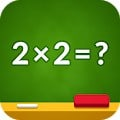# Multiplication Table IQ APK

The Multiplication and Division Tables" application was built based on algorithms that adapt the questions to the child's current skills.

The application adapts the learning intensity, focusing on the mathematical operations the child has the most difficulty with.

A special algorithm showing the learning progress and placing emphasis on the mathematical operations that are more difficult to learn.

Intelligent repetition system.

The learning process is visualized using progress stars. This allows you to check both the learning progress for individual activities as well as the overall progress. The algorithm separately calculates the progress for multiplication and division tables. Additionally, it adapts to the currently selected ranges of results and ranges of numbers used in the mathematical operations. Both settings and the learning mode can be changed in the application's options.

A modern learning method for children.

The learning process is divided into numbered lessons, so that a parent can have control over the number of lessons completed by their child.

Minor fixes